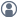###Show Posts

This section allows you to view all posts made by this member. Note that you can only see posts made in areas you currently have access to.

### Topics - fangbiaolee

Pages: 
1
##### General Questions and Answers / AIMD trajectory to MTP training data
« on: June 22, 2022, 04:28 »
Dear ATK-Staff/developer:
when I loaded the AIMD trajectory object which was precalculated：

# Load another Trajectory object with precalculated data.
training_sets.append(
TrainingSet(trajectory_training_data_input,
# sample_size=100,
calculator=calculator,
recalculate_training_data=False)

I encountered the following problem:

Traceback (most recent call last):
File "test3.py", line 58, in <module>
IndexError: list index out of range
application called MPI_Abort(MPI_COMM_WORLD, 1) - process 31

The  measurements of MD_trajectory are shown as follow: kinetic_energy, potential_energy, volume, temperature, pressure and stress.
And that are differs from the “energy”, “force” and “stress”required for the training set. Moreover,  there is no option for “force” in the measurements  in AIMD simulation.

So how do I solve this problem so that I can make use of the AIMD data that has been calculated before.

2
##### General Questions and Answers / MTP——“No new candidates found in active learning MD”
« on: June 18, 2022, 09:08 »
Dear ATK-Staff/developer,

I used the AIMD  trajectory as the initial training set to generate the MTP potential, although I lowered the basis set of the MTP many times, the same problem still occurs--"  No new candidates found in active learning MD", is there any way to solve such a problem, or my initial training set has question?

part of the code:

training_set = TrainingSet(trajectory,
sample_size=1000,
calculator=calculator)

fitting_parameters = MomentTensorPotentialFittingParameters(
basis_size=200,
constant_terms=constant_terms,
mtp_filename='mtp_potential.mtp',
forces_cap= 100.0 * eV / Angstrom,
non_linear_coefficients_parameters=non_linear_coefficients_parameters,
)

WARNING: The condition number of the optimized training matrix is            |
|          5.09e+13.This could lead to accuracy problems when calculating the  |
|          extrapolation grade.Consider reducing the number of MTP basis       |
|          functions or adding more diverse training configurations until the  |
|          condition number is lower.                                          |

Pages: